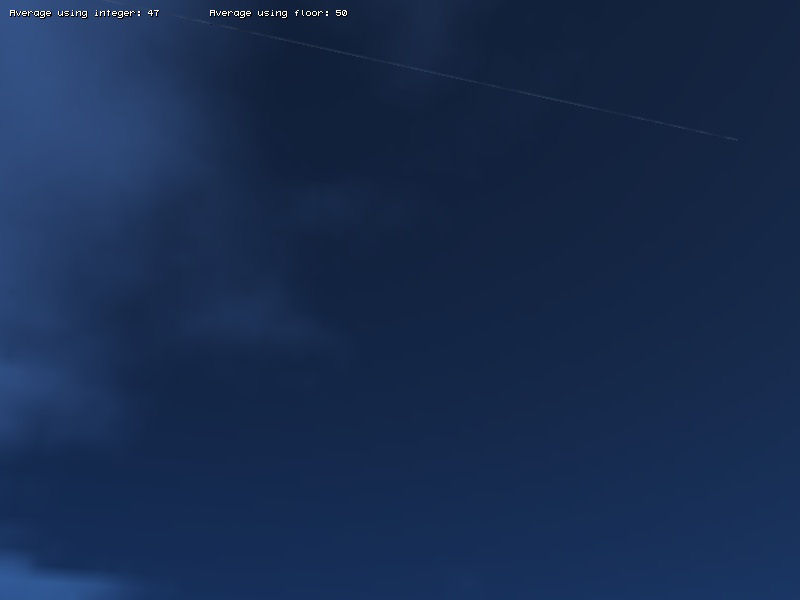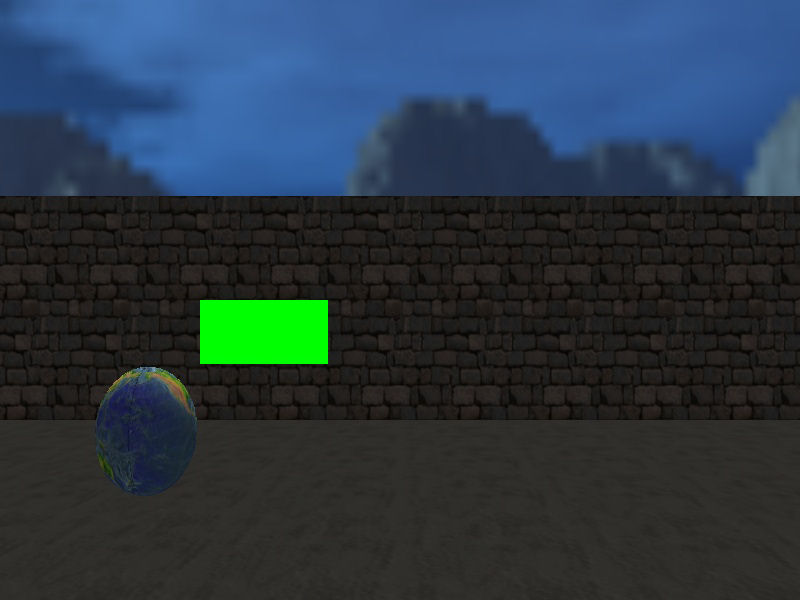New engine features
 USB I/O Control   USB I/O modules (such as relays) can now be controlled using the new usb functions. Modules with up to 16 relays are available for purchase and can be user to control pretty much any external device you can think of (light bulbs, TV sets, etc) from within the engine.   #include #include #include   // control USB relay with the  and  keys function main() { usb_open(0); while(1) {    if(key_1)      usb_out(1); // relay 1    else if(key_2)      usb_out(2); // relay 2    else      usb_out(0);    wait(1); } }   function on_exit_event() { usb_close(); }     floor(x)   This new function computes the largest integer that is less than or equal to x; it generates an equal distribution of the values, while integer( ) converts more values to 0.   var average1 = 0; var average2 = 0;   function compute_startup() {        random_seed(0); // generate really random numbers        int i;        for (i = 0; i < 100; i++) // generate 100 integer numbers that have random values        {                average1 += integer(random(100)) / 100; // computes the average using integer                average2 += floor(random(100)) / 100; // computes the average using floor                        }                 }   PANEL* output_pan = {        layer = 15;        digits(10, 10, "Average using integer: %.f", *, 1, average1);        digits(210, 10, "Average using floor: %.f", *, 1, average2);                 flags = SHOW; }bmap_createblack(size_x, size_y, format);   Creates a black bmap with given dimensions that can be filled with a color.   PANEL* my_pan;   function bitmap_startup() {        wait (-5); // wait for 5 seconds        BMAP* my_bitmap = bmap_createblack(128, 64, 8); // create a black bitmap of 128 x 64 pixels, 8 bits (256 colors) per pixel        bmap_fill (my_bitmap, vector(0, 255, 0), 80); // fill the bitmap with a green color, alpha = 80        my_pan = pan_create("bmap = NULL;", 10); // create a panel at runtime        my_pan.bmap = my_bitmap; // and assign it our previously created bitmap        my_pan.pos_x = 200; // place it at x = 200, y = 300 on the screen        my_pan.pos_y = 300;        my_pan.flags |= SHOW; // and then make it visible         }